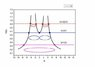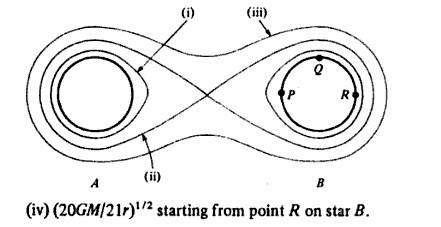# Gravitational potential of two equal stars

• benf.stokes
In summary, the gravitational potential of the double star system is the sum of the potential of the single stars. The potential in -GM/r units is easy to calculate and sketch.f

## Homework Statement

Two equal spherically symmetrical stars, A and B, each of mass M and radius r have their centers 6r apart. Make a sketch showing the lines on which the gravitational potential takes the values:
1. $$\frac{-10GM}{11r}$$

2. $$\frac{-2GM}{3r}$$

3. $$\frac{-GM}{3r}$$

Accurate plotting is not required but the general shape of the lines must be clear
4. What is the minimum velocity with which gas can be emitted from the surface of B and still be captured by A? Ignore any effects due to the motion of the stars

## Homework Equations

U = $$\frac{-GM}{R}$$

## The Attempt at a Solution

I can't even get started.

Thanks

Last edited:
The potential of the double-star system is the sum of the potential of the single stars:
V=-GM(1/R1+1/R2),
where R1 is the distance from the star on the left and R2 is the distance from the star on the right. We can calculate the potential in -GM/r units, to make sketching equipotential curves easy.

In case of a lonely star, the equipotential curves are circles around the star. These circles will be somewhat distorted in the presence of the other star but they still are a pair of closed curves when we are at the close vicinity of one star.

It is easy to plot the potential values along the line which connects the stars. If the origin is the middle point between the starts, the potential function is V(x) = 1/x+1/(6-x) (multiplied by -GM/r). Drawing horizontal lines at the height of the given potential values, one can find the points where the equipotential curves cross the x axis.
In the middle, the potential of both stars is the same, the equipotential curves around both stars touch here. If the magnitude of the potential is lower than 2/3 the equipotential curve crosses the x-axis outside the two-star system, and encloses both stars.

ehild

#### Attachments

•twostars.jpg
15.9 KB · Views: 422
Thanks for the help but isn't there any general way to determine the equipotential surfaces of the two stars? I happen to have the answer to the problem (I just can't figure it out) and it is like this:Look at the graph ehild has shown and simply look at it as though it were top-down.

You can calculate the equipotential curves, but it was not required.

For r=1, the equation for these curves is :

$$\frac{1}{\sqrt{(x+3)^2+y^2}}+\frac{1}{\sqrt{(x-3)^2+y^2}}=C$$

But it is not easy to plot them.

ehild